# Mock exam paper E Paper 1 Marking Scheme

Pre-Junior Cert Examination

Mathematics

Paper 1

Higher Level

Marking Scheme

1 of 23

Question 1 (5 mins)

1. Ellen is going to Australia on holiday and wants to exchange Euro for Australian dollars. She has BC2000. The exchange rate is BC1 = A\$1.44 and she has to pay 5% commission. How much money will Ellen get?
 BC1 = A\$1:44 BC2000 = 2000 1:44 = A\$2880 Less 5% commission 2880 5 = 144 100 2880 144 = A\$2736
1. Ellen has A\$300 when she comes home. If the exchange rate is now AU\$1.40 = BC1 and she still has to pay 5% commission, how much Euro will she get back, to the nearest cent?
 A\$1:40 = BC1 A\$300 = 300 = 214:29 1:40 Less 5% commission 214:29 5 = 10:71 100 214:29 10:71 = BC203:58

2 of 23

Question 2 (5 mins)

1. Fill out the following table with a tick to indicate all sets a number belongs to.
 Number N Z Q R 2 X X X X p X 5 3=2 X X -7.3 X X X
1. Explain why you have placed 3=2 and into the sets you have.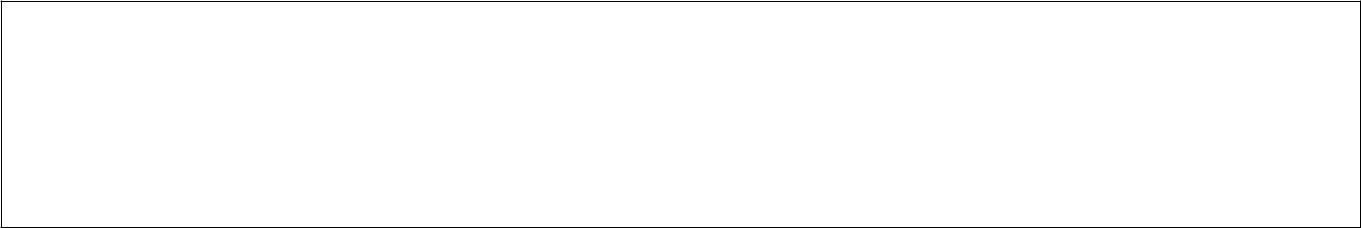3=2 is a rational number because it is a simple fraction. It is real because it is not imaginary.

is real because it is not imaginary but doesn't t the other categories because it cannot be expressed as a fraction and it is not a whole number.

3 of 23

Question 3 (10 mins)

1. 70 students were surveyed to nd out who participated in certain sports. The results showed that 35 played football, 13 did swimming and 10 did both.

How many students didn't participate in either sport?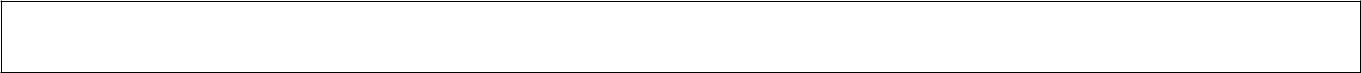70 - (35 + 13 + 10) = 12

1. Draw a Venn diagram to represent all the above information.

R

12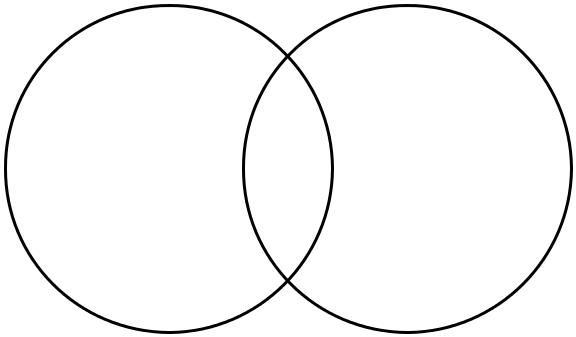F S

35 10 13

1. What is the probability of a randomly chosen student playing both sports. Write your answer as a fraction.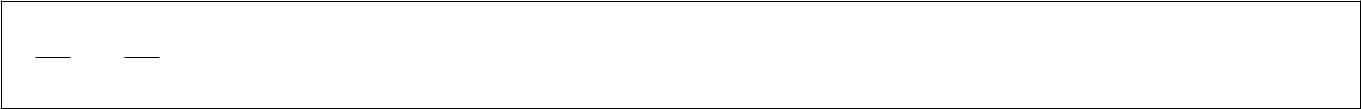1270 = 356

1. How many students are in both football and swimming together or one of the sports on their own?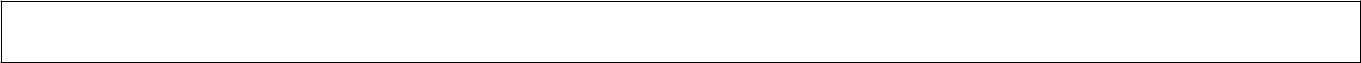35 + 10 + 13 = 58

4 of 23

Question 4 (5 mins)

Factorise each of the following expressions fully.

1. 3x2 + 9x3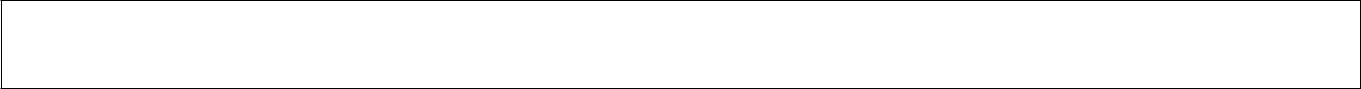3x2 (1 + 3x)

1. 9a2 121b2(3z + 11y) (3x 11y)

1. x2 xy 2x + 2y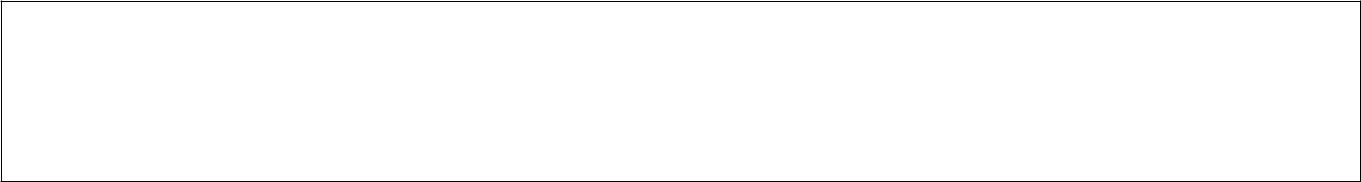x(x y) y(x 2)

(x y)(x 2)

5 of 23

Question 5 (10 mins)

Below is a graph showing 3 cheetahs running for 100 seconds.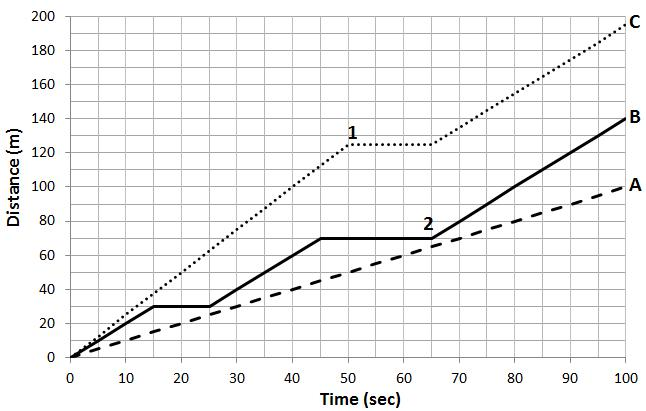1. Which cheetah ran the farthest and how far did they run? Runner C ran the farthest at 195 m.
2. How lo...